# MCQ Questions for Class 12 Maths Chapter 4 Determinants with Answers

Students can access the NCERT MCQ Questions for Class 12 Maths Chapter 4 Determinants with Answers Pdf free download aids in your exam preparation and you can get a good hold of the chapter. Use MCQ Questions for Class 12 Maths with Answers during preparation and score maximum marks in the exam. Students can download the Determinants Class 12 MCQs Questions with Answers from here and test their problem-solving skills. Clear all the fundamentals and prepare thoroughly for the exam taking help from Class 12 Maths Chapter 4 Determinants Objective Questions.

## Determinants Class 12 MCQs Questions with Answers

Students are advised to solve the Determinants Multiple Choice Questions of Class 12 Maths to know different concepts. Practicing the MCQ Questions on Determinants Class 12 with answers will boost your confidence thereby helping you score well in the exam.

Explore numerous MCQ Questions of Determinants Class 12 with answers provided with detailed solutions by looking below.

Question 1.
If $$\left|\begin{array}{rr} x & 2 \\ 18 & x \end{array}\right|$$ = $$\left|\begin{array}{rr} 6 & 2 \\ 18 & 6 \end{array}\right|$$, then x is equal to
(a) 6
(b) ±6
(c) -6
(d) 6, 6

Question 2.
Let A be a square matrix of order 3 × 3. Then |kA| is equal to
(a) k|A|
(b) k²|A|
(c) k³|A|
(d) 3k|A|

Question 3.
Which of the following is correct?
(a) Determinant is a square matrix
(b) Determinant is a number associated to a matrix
(c) Determinant is a number associated to a square matrix
(d) None of these.

Answer: (c) Determinant is a number associated to a square matrix

Question 4.
If area of triangle is 35 sq. units with vertices (2, -6), (5, 4) and (k, 4). Then k is
(a) 12
(b) -2
(c) -12, -2
(d) 12, -2.

Question 5.
If A = $$\left[\begin{array}{lll} a_{11} & a_{12} & a_{13} \\ a_{21} & a_{22} & a_{23} \\ a_{31} & a_{32} & a_{33} \end{array}\right]$$ and Aij is co-factors of aij, then A is given by
(a) a11A31 +a12A32 + a13A33
(b) a11A11 + a12A21 + a13A33
(c) a21A11 + a22A12 + a23A13
(d) a11A11 + a21A21 + a31A31

Answer: (d) a11A11 + a21A21 + a31A31

Question 6.
Let A be a non-singular matrix of order 3 × 3. Then |adj. A| is equal to
(a) |A|
(b) |A|²
(c) |A|³
(d) 3|A|

Question 7.
If A is any square matrix of order 3 x 3 such that |a| = 3, then the value of |adj. A| is?
(a) 3
(b) $$\frac { 1 }{3}$$
(c) 9
(d) 27

Question 8.
If A is an invertible matrix of order 2, then det (A-1) is equal to
(a) det (A)
(b) $$\frac { 1 }{det(A)}$$
(c) 1
(d) 0

Answer: (b) $$\frac { 1 }{det(A)}$$

Question 9.
If a, b, c are in A.P., then determinant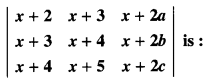(a) 0
(b) 1
(c) x
(d) 2x

Question 10.
If x, y, z are non-zero real numbers, then the inverse of matrix A = $$\left[\begin{array}{lll} x & 0 & 0 \\ 0 & y & 0 \\ 0 & 0 & z \end{array}\right]$$ is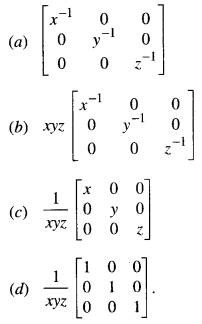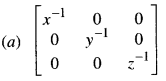Question 11.
Let A =where 0 ≤ θ ≤ 2π then
(a) Det (A) = 0
(b) Det (A) ∈ (2, ∞)
(c) Det (A) ∈ (2, 4)
(d) Det (A) ∈ [2, 4]

Answer: (d) Det (A) ∈ [2, 4]

Question 12.
If $$\left|\begin{array}{rr} 2x & 5 \\ 8 & x \end{array}\right|$$ = $$\left|\begin{array}{rr} 6 & -2 \\ 7 & 3 \end{array}\right|$$, then value of ‘x’ is
(a) 3
(b) ±3
(c) ±6
(d) 6

Question 13.
Let Δ = $$\left|\begin{array}{lll} \mathbf{A} x & x^{2} & 1 \\ B y & y^{2} & 1 \\ C z & z^{2} & 1 \end{array}\right|$$ and Δ1 = $$\left|\begin{array}{rrr} \mathbf{A} & \mathbf{B} & \mathbf{C} \\ \boldsymbol{x} & \boldsymbol{y} & z \\ z \boldsymbol{y} & z x & x y \end{array}\right|$$, then
(a) Δ1 = -Δ
(b) Δ ≠ Δ1
(c) Δ – Δ1 = 0
(d) None of these

Answer: (c) Δ – Δ1 = 0

Question 14.
If x, y ∈R, then the determinant: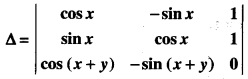lies in the interval
(a) [-√2, √2]
(b) [-1, 1]
(c) [√2, 1]
(d) [-1, √2]

Question 15.
The area of a triangle with vertices (-3, 0), (3, 0) and (0, k) is 9 sq. units. The value of ‘k’ will be:
(a) 9
(b) 3
(c) -9
(d) 6.

Question 16.
If A, B and C are angles of a triangle, then the determinant:
$$\left|\begin{array}{ccc} -1 & \cos C & \cos B \\ \cos C & -1 & \cos A \\ \cos B & \cos A & -1 \end{array}\right|$$ is equal to
(a) 0
(b) -1
(c) 1
(d) None of these.

Question 17.
Let A be a square matrix all of whose entries are integers. Then which of the following is true?
(a) If det A = ± 1, then A-1 need not exist
(b) If det A = ± 1, then A-1 exists but all entries are not necessarily integers.
(c) If det A ≠ ± 1, then A-1 exists and all its entries are non-integers
(d) If det A = ± 1, then A-1 exists and all its entries are integers.

Answer: (d) If det A = ± 1, then A-1 exists and all its entries are integers.
Hint:
Since each entry of A is an integer,
∴ co-factor of each entry is also an integer.
Hence, each entry of the adjoint is an integer.
Also det A = ± 1 and A-1 = $$\frac { 1 }{det(A)}$$ (adj A).
Hence, all entries of A-1 are integers.

Question 18.
The number of values of ‘k’ for which the linear equations:
4x + ky + 2z = 0
kx + 4y + z = 0
2x + 2y + z = 0
possesses a non-zero solution is
(a) 3
(b) 2
(c) 1
(d) zero.

Hint:
The system possesses non-zero solution
If $$\left|\begin{array}{lll} 4 & k & 2 \\ k & 4 & 1 \\ 2 & 2 & 1 \end{array}\right|$$ = 0
If 4(4 – 2) + k (k – 2) + 2(2k – 8) = 0
if = 8 – k² + 2k + 4k – 16 = 0
if k² – 6k + 8 = 0
if (k – 2)(k – 4) = 0
if k = 2 or 4
k = 2.

Question 19.
If A = $$\left[\begin{array}{lll} 1 & \alpha & 3 \\ 1 & 3 & 3 \\ 2 & 4 & 4 \end{array}\right]$$ is the adjoint of a 3 × 3 matrix A and |A| = 4 then α is equal to
(a) 11
(b) 5
(c) 0
(d) 4

Hint:
= |A|² = 4²
= 16
⇒ 1. (12 – 12) -α (4 – 6)+ 3(4 – 6) = 16
⇒ 2α – 6 = 16
⇒ 2α = 22.
Hence, α = 11.

Question 20.
If α, ß ≠ 0 and f(x) = α” + ß” and= k (1 – α)²(1 – ß)²(α – ß)², then ‘4k’ is equal to:
(a) $$\frac { 1 }{αß}$$
(b) 1
(c) -1
(d) αß

Hint: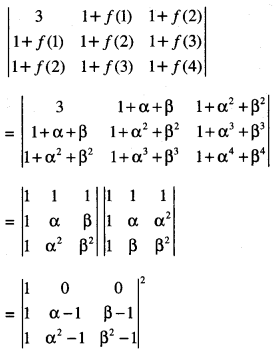= [(α – 1) (ß² – 1) – (α² – 1) (ß – 1)]²
= (α – 1)²(ß – 1)²(α – ß)2.
Hence, k = 1

Question 21.
The system of linear equations:
x + λy – z = 0
λr – y – z = 0
x + y – λz = 0
has a non-trivial solution for
(a) Exactly one value of λ
(b) Exactly two values of λ
(c) Exactly three values of λ
(d) Infinitely many values of λ.

Answer: (c) Exactly three values of λ
Hint:
The system AX = O has non-trivial solution if det A = 0
i.,e if $$\left|\begin{array}{rrr} 1 & \lambda & -1 \\ \lambda & -1 & -1 \\ 1 & 1 & -\lambda \end{array}\right|$$ = 0
⇒ (1)(λ + 1) -λ(-λ² + 1) + (-1)(λ + 1) = 0
⇒ λ + 1 + λ³ – λ – λ – 1 = 0
⇒ λ³ – λ = 0
⇒ λ(λ² – 1) = 0
⇒ λ = 0, 1, -1.
Hence, λ = -1, 0, 1.

Question 22.
Let on be a complex number such that 2ω + 1 = z, where z = √-3
If $$\left|\begin{array}{ccc} 1 & 1 & 1 \\ 1 & -\omega^{2}-1 & \omega^{2} \\ 1 & \omega^{2} & \omega \end{array}\right|$$ = 3k the k is equal to
(a) -1
(b) 1
(c) z
(d) -z

Hint: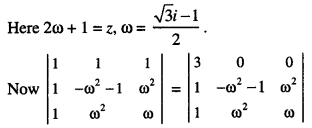[Operating R1 → R1 + R2 + R3]
= 3[- ω (ω² + 1) – ω4]
= 3[- ω³ – ω – ω] = 3[- 1 – 2ω]
= – 3(1 + 2ω) = – 3z.
Thus 3k = – 3z.
Hence, k = -z.

Question 23.
If Sis the set of distinct values of ‘h’ for which the following system of linear equations:
x + y + z = 1,
x + ay + z = 1,
ax + by + z = 1
has no solution, then S is
(a) a finite set containing two or more elements
(b) a singleton
(c) an empty set
(d) an infinite set.

Hint:
D = $$\left|\begin{array}{lll} 1 & 1 & 1 \\ 1 & a & 1 \\ a & b & 1 \end{array}\right|$$ = 0
⇒ a – 1
⇒ x + y + z = 1
and x + by + z = 0.
The planes are parallel
⇒ b = 1.
Hence, S is a singleton.

Question 24.
If A = $$\left[\begin{array}{rr} 2 & -3 \\ -4 & 1 \end{array}\right]$$, then adj. (3A² + 12A) is equal toHint: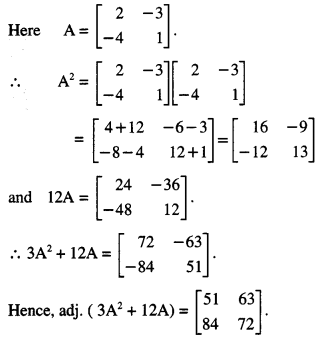Question 25.
If the system of linear equations:
x + ky + 3z = 0
3x + ky – 2z = 0
2x + 4y – 3z = 0
has a non zero solution (x, y, z), then $$\frac {xz}{y^2}$$ is equal to:
(a) -10
(b) 10
(c) -30
(d) 30

Hint:
The given system has non-zero solution
⇒ $$\left|\begin{array}{ccc} 1 & k & 3 \\ 3 & k & -2 \\ 2 & 4 & -3 \end{array}\right|$$
⇒ 1(-3k + 8)-k (-9 + 4) + 3 (12 – 2k) = 0
⇒ 44 – 4k = 0
⇒ k = 11
Let z = λ
Thus x + 11 y = -3λ
and 3x + 11 y = 2λ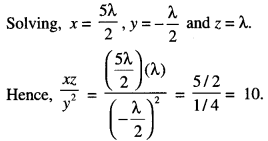Fill in the blanks

Question 1.
If $$\left|\begin{array}{ll} x & 2 \\ 8 & x \end{array}\right|$$ = $$\left|\begin{array}{ll} 3 & 2 \\ 9 & 6 \end{array}\right|$$, then the value of x is ……………..

Hint:
$$\left|\begin{array}{ll} x & 2 \\ 8 & x \end{array}\right|$$ = $$\left|\begin{array}{ll} 3 & 2 \\ 9 & 6 \end{array}\right|$$
⇒ x² – 16 = 18 – 18
⇒ x² = 16
⇒ x = ±4.

Question 2.
Let A be a 3 x 3 determinant and |A| = 7. Then the value of |2A| is ……………….

Hint:
|2A| = 2³ |A| = 8 x 7 = 56.

Question 3.
If A = $$\left[\begin{array}{ll} 1 & 2 \\ 4 & 2 \end{array}\right]$$ Then the value of k = ……………
if |2A| = k|A|

Hint:
if |2A| = 2²|A| = 4|A|
k = 4

Question 4.
If A is a skew-symmetric matrix of order 3, then det A = ……………..

Question 5.
The value of $$\left[\begin{array}{ccc} 102 & 18 & 36 \\ 1 & 3 & 4 \\ 17 & 3 & 6 \end{array}\right]$$ is ……………..

Hint:
Δ = 6$$\left|\begin{array}{ccc} 17 & 3 & 6 \\ 1 & 3 & 4 \\ 17 & 3 & 6 \end{array}\right|$$ = 6(0) = 0

Question 6.
If Δ = $$\left|\begin{array}{ll} 1 & a \\ 1 & b \end{array}\right|$$, then minor of ‘b’ is ………………

Question 7.
Minor of ‘d’ is = $$\left|\begin{array}{ll} a & c \\ b & d \end{array}\right|$$, is ………………

Question 8.
A square matrix A has inverse if and only if A is ………………

Question 9.
Co-factor of ‘b’ in $$\left|\begin{array}{ll} a & c \\ b & d \end{array}\right|$$ is ……………

If Δ = $$\left|\begin{array}{lll} 1 & 2 & 3 \\ 2 & 0 & 1 \\ 5 & 3 & 8 \end{array}\right|$$ then minor of a22 is …………….